# GSEB Solutions Class 10 Maths Chapter 12 Areas Related to Circles Ex 12.2

Gujarat Board GSEB Solutions Class 10 Maths Chapter 12 Areas Related to Circles Ex 12.2 Textbook Questions and Answers.

## Gujarat Board Textbook Solutions Class 10 Maths Chapter 12 Areas Related to Circles Ex 12.2

Question 1.
Find the area of a sector of a circle with radius 6 cm, if angle of the sector is 60°.
Solution:
Here ∠AOB = 60°
and radius r = 6 cm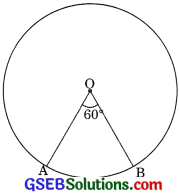Area of sector = $$\frac{\pi r^{2} \theta}{360^{\circ}}$$
= $$\frac{\frac{22}{7} \times 6 \times 6 \times 60^{\circ}}{360^{\circ}}$$
= $$\frac {132}{7}$$ cm2

Question 2.
Find the area of a quadrant of a circumference is 22 cm. circle whose
Solution:
Here circumference of a circle = 22 cm
2πr = 22
⇒ 2 x $$\frac {22}{7}$$r = 22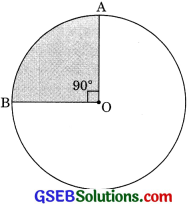⇒ $$\frac{22 \times 7}{2 \times 22}$$
⇒ r = $$\frac {7}{2}$$ cm
Area of quadrant = $$\frac {1}{4}$$πr2
= $$\frac {1}{4}$$ x $$\frac {22}{7}$$ x ($$\frac {7}{2}$$)2
= $$\frac {1}{4}$$ x $$\frac {22}{7}$$ x $$\frac {7}{2}$$ x $$\frac {7}{2}$$
= $$\frac {77}{8}$$ cm2Question 3.
The length of the minute hand of a clock is 14 cm. Find the area swept by the minute hand in 5 minutes.
Solution:
Length of minute hand = 14 cm
Radius of circle made by minute hand = Length of minute hand
r = 14cm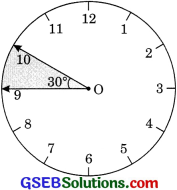Angle moved in 1 minute = 6°
So angle moved in 5 minutes = 5 x 6° = 30°
Area swept by minute hand in 5 minutes
= $$\frac{\frac{22}{7} \times 14^{2} \times 30^{\circ}}{360^{\circ}}$$
= $$\frac{22 \times 14 \times 14 \times 30}{7 \times 360}$$
= $$\frac {154}{3}$$ cm2

Question 4.
A chord of a circle of radius 10 cm subtends a right angle at the centre. Find the area of the corresponding:
(i) minor segment (CBSE 2016, 2017)
(ii) major sector. (Use π = 3.14)
Solution:
(i) Here, radius = 10 cm and θ = 90°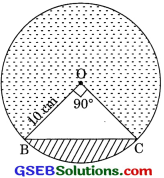Area of minor segment = $$\frac{\pi r^{2} \theta}{360^{\circ}}$$ – ar ΔBOC
= $$\frac{\frac{22}{7} \times 10 \times 10 \times 90^{\circ}}{360^{\circ}}$$ – $$\frac {1}{2}$$ x 10 x 10
= $$\frac {22}{7}$$ x 25 – 50 = $$\frac {200}{7}$$ = 28.57 cm2(ii) Area of major sector
= $$\pi r^{2}-\frac{\pi r^{2} \theta}{360^{\circ}}$$
= πr2$$\left[1-\frac{\theta}{360}\right]$$
= $$\frac {22}{7}$$ x 102$$\left[1-\frac{90}{360}\right]$$
= $$\frac {22}{7}$$ x 100 $$\left[1-\frac{1}{4}\right]$$
= $$\frac{2200}{7}$$ x $$\frac{3}{4}$$ = 235.71 cm2

Question 5.
In a circle of radius 21 cm, an arc subtends an angle of 60° at the center. Find:
(i) the length of the arc
(ii) area of the sector formed by the arc
(iii) area of the segment formed by the corresponding chord.
Solution:
(i) Here, r = 21 cm
and ∠BOC = 60°
Length of arc = $$\frac{2 \pi r \theta}{360^{\circ}}$$
= $$\frac{2 \times \frac{22}{7} \times 21 \times 60^{\circ}}{360^{\circ}}$$ = 22 cm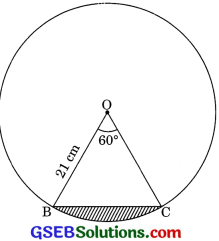(ii) Area of sector formed by arc = $$\frac{2 \pi r \theta}{360^{\circ}}$$
= $$\frac{\frac{22}{7} \times 21 \times 21 \times 60^{\circ}}{360^{\circ}}$$ = 231 cm2

(iii) OAB is isosceles with vertical angle 600. That means it is an equilateral triangle.
∴ ar(ΔOBC) = $$\frac{\sqrt{3}}{4}$$ a2
= $$\frac{\sqrt{3}}{4}$$ (21)2
= $$\frac{44 \sqrt{3}}{4}$$ cm2
Area of segment = Area of sector – ar (ΔOBC)
= (231 – $$\frac{44 \sqrt{3}}{4}$$) cm2Question 6.
A chord of a circle of radius 15 cm subtends an angle of 60° at the centre. Find the areas of the corresponding minor and major segments of the circle. (Use π = 3.14 and $$\sqrt{3}$$ = 1.73)
Solution:
Here r = 15 cm
θ = 60°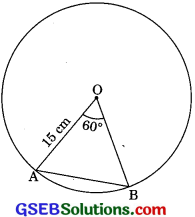Area of ΔOBC = $$\frac{44 \sqrt{3}}{4}$$ a2
= $$\frac{44 \sqrt{3}}{4}$$ (15)2
= $$\frac{225 \times 1.73}{4}$$ = 97.3125 cm2 ………(1)
Area of sector = $$\frac{\pi r^{2} \theta}{360^{\circ}}$$
= $$\frac{3.14 \times 15 \times 15 \times 60^{\circ}}{360^{\circ}}$$
= 1.57 x 75 = 117.75 cm2 ………(2)
Area of minor segment = Area of sector – ar (ΔOAB)
= 117.75 cm2 – 97.3125 cm2
= 20.4375 cm2
Area of major segment = nr2 – 20.4375
= 3.14 x 15 x 15 – 20.4375
= 706.5 – 20.4375
= 686.0625 cm2Question 7.
A chord of circle of radius 12 cm subtends an angle of 120° at the centre. Find the area of the corresponding segments of the circle.
Solution:
Here r = 12 cm
and θ = 120°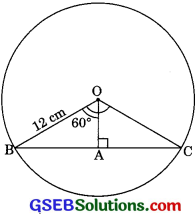Draw OA ⊥ BC
In right ΔOAB
cos 60° = $$\frac {OA}{OB}$$
⇒ $$\frac {1}{2}$$ = $$\frac {OA}{12}$$
OA = 6cm
Again,
sin 60° = $$\frac {AB}{OB}$$
⇒ $$\frac{\sqrt{3}}{2}$$ = $$\frac {AB}{12}$$
⇒ AB = $$\frac{12 \sqrt{3}}{2}$$ = 6$$\sqrt{3}$$
BC = 2 x 6$$\sqrt{3}$$ = 12$$\sqrt{3}$$ cm
Area of ΔOBC = $$\frac {1}{2}$$ x BC x OA
= $$\frac {1}{2}$$ x 12$$\sqrt{3}$$ x 6
= 36$$\sqrt{3}$$
Area of corresponding segment = Area of sector – ar (ΔOBC)
= $$\frac{\pi r^{2} \theta}{360}$$ – 36$$\sqrt{3}$$
= $$\frac{3.14 \times 12 \times 12 \times 120^{\circ}}{360^{\circ}}$$ – 36 x 1.73
= 150.72 – 62.28
= 8844 cm2Question 8.
A horse is tied to a leg at one corner of a square-shaped grass field of side 15 m by means of a 5 m long rope (see figure). Find:
(i) The area of that part of the field in which the horse can graze.
(ii) The increase in the grazing area of the rope were 10 m long instead of 5 m. (Use π = 3.14)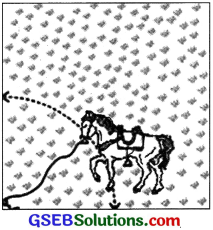Solution:
(i) Horse can graze field in the form of a quadrant part of the circle.
r1 = 5 m
and θ = 90°
Area of field that can be grazed by horse
= $$\frac {1}{4}$$πr21
= $$\frac {1}{4}$$ x 3.14 x 5 x 5 = 19.625 m2

(ii) Now radius r2 = 10 m
Area of field can be grazed by horse
= $$\frac {1}{4}$$πr22
= $$\frac {1}{4}$$ x 3.14 x 10 x 10
= 78.5 m2
Increase of area in the grazing field
= 78.5 – 19.625
= 58.875 m2Question 9.
A brooch is made with silver wire in the form of a circle with diameter 35 mm. The wire is also used in making 5 diameters which divide the circle into 10 equal sectors as shown in figure find: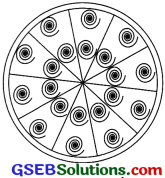(i) the total length of silver wire required
(ii) the area of each sector of the brooch.
Solution:
(i) Diameter of circle = 35 mm
Radius r = $$\frac {35}{2}$$ mm
Circumference of cirde = 2πr
= 2 x $$\frac {22}{7}$$ x $$\frac {35}{2}$$ = 110 mm
Length of 5 diameters = 35 x 5 = 175 mm
Total length of silver wire required = 110 + 175 = 285 mm

(ii) r = $$\frac {35}{2}$$ mm
and θ = $$\frac {360°}{10}$$
θ = 36°
Area of each sector of the brooch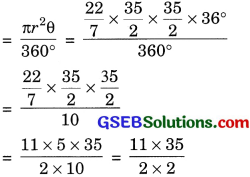= $$\frac {385}{4}$$ mm2 or 96.25 mm2Question 10.
An umbrella has 8 ribs which are equally spaced (see figure). Assuming umbrella to be a flat circle of radius 45 cm. Find the area between the two consecutive ribs of the umbrella.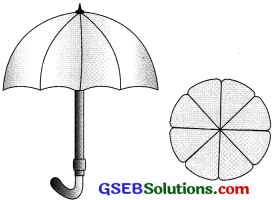Solution:
Here
r = 45cm
and θ = $$\frac {360°}{8}$$ = 45°
Area between two consecutive ribs of the umbrella
= $$\frac{\pi r^{2} \theta}{360^{\circ}}$$
= $$\frac{\frac{22}{7} \times 45 \times 45 \times 45}{360}$$
= $$\frac{22275}{7 \times 4}$$
= $$\frac{22275}{28}$$ cm2 or 795.54 cm2

Question 11.
A car has two wipers which do not overlap. Each wiper has a blade of length 25 cm sweeping through angle of 115°. Find the total area cleaned at each sweep of the blades.
Solution:
Here
r = 25 cm
θ = 115°
Total area cleaned at each sweep of the blades
= 2 x $$\frac{\pi r^{2} \theta}{360^{\circ}}$$
= $$\frac{2 \times \frac{22}{7} \times 25 \times 25 \times 115^{\circ}}{360^{\circ}}$$
= $$\frac{3162500}{7 \times 360}$$
= $$\frac{158125}{126}$$cm2
= 158125 cm2 or 1254.96 cm2Question 12.
Two warn ships for underwater rocks a lighthouse spreads a red coloured light over a sector of angle 80° to a distance of 16.5 km. Find the area of the sea over which the ships are warned. (Use π = 3.14)
Solution:
Here r = 16.5 km
and θ = 80°
The area of the sea over which the ships are warned
= $$\frac{\pi r^{2} \theta}{360^{\circ}}$$
= $$\frac{3.14 \times 16.5 \times 16.5 \times 80^{\circ}}{360^{\circ}}$$
= 189.97 km2

Question 13.
A round table cover has six equal designs as shown in figure. If the radius of the cover is 28 cm, find the cost of making the design at the rate of ₹ 0.35 per cm2. (Use $$\sqrt{3}$$ = 1.7)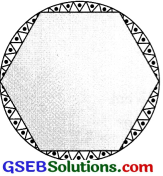Solution:
Here r = 28 cm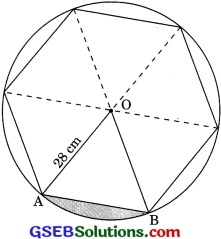θ = $$\frac{360^{\circ}}{6}$$
⇒ θ = 60°
r = 28 cm
⇒ ΔOAB is an equilateral triangle with side 28 cm.
Area of ΔOAB = $$\frac{\sqrt{3}}{4}$$ a2
= $$\frac{\sqrt{3}}{4}$$ (28)2
= 196 $$\sqrt{3}$$ cm2 = 333.2 cm2Area of sector = $$\frac{\pi r^{2} \theta}{360^{\circ}}$$
= $$\frac{\frac{22}{7} \times 28 \times 28 \times 60^{\circ}}{360^{\circ}}$$
= $$\frac{22 \times 28 \times 28 \times 60}{7 \times 360}$$
= 410.67 cm2
Area of one segment
= Area of sector – Area of ΔOAB
= 410.67 – 333.2
= 77.47 cm2
Area of six segment or design
= 6 x 77.47 = 464.82
Cost of making six designs
= 464.82 x ₹ 0.35 = ₹ 162.68

Question 14.
Tick the correct answer in the following: Area of a sector of angle p (in degrees) of a circle with radius R is
(a) $$\frac{p}{180}$$ x 2πR
(b) $$\frac{p}{180}$$ x πR2
(c) $$\frac{p}{360}$$ x 2πR2
(d) $$\frac{p}{720}$$ x 2πR2
Solution:
Here θ = p°
Then area of sector of a circle = $$\frac{\pi r^{2} \theta}{360^{\circ}}$$
= $$\frac{\pi r^{2} \theta}{360^{\circ}}$$
= $$\frac{2 \times \pi \mathrm{R}^{2} p}{2 \times 360}$$
= $$\frac{2 \pi \mathrm{R}^{2} p}{720}$$
Hence, correct answer is (d) $$\frac{p}{720}$$ x 2πR2